# Search

## Definitions

### Minimax

Minimax is a decision rule used for minimizing the possible loss of the worst case (maximum loss) scenario.

## Tree search

### alpha-beta pruning

Alpha-beta pruning is a search algorithm that seeks to decrease the number of nodes that are evaluated by the minimax algorithm in its search tree.

It stops completely evaluating a move when at least one possibility has been found that proves the move to be worse than a previously examined move. Such moves need not to be evaluated further. When applied to a standard minimax tree, it returns the same move as minimax would, but prunes away branches that cannot possibly influence the final decision.

It is an adverserial search algorithm.

#### Psuedo-code

def alpha_beta(node, depth, alpha, beta, is_maximizing_player):
if depth == 0 or node.is_terminal_node:
return node
if is_maximizing_player:
v = - np.inf
for child in node.children:
v = max(v, alpha_beta(child, depth - 1, alpha, beta, False))
a = max(alpha, v)
if beta <= alpha:
break
return v
else:
v = np.inf
for child in node.children:
v = min(v, alpha_beta(child, depth - 1, alpha, beta, True))
beta = min(beta, v)
if beta <= alpha:
break
return v


### Monte Carlo Tree Search (MCTS)

#### Overview

• Each node holds:
•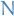- the number of times this game-state has been visited
•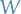- the value of this game-state, which is computed by some function and the accumulated value of it's child-nodes#### Algorithm

1. Start at some root state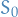2. Perform action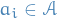to obtain the"child-state"
3. Compute values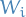for eachchild
4. Update the parent statewith the number of times we played in this state, i.e.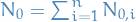where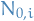denotes theof the each of the child states
5. Unroll child-nodes in a smiliar way, always propagating the counts and values up the tree.
6. When some terminating condition has been reached, choose the optimal child of the root stateas the next move.